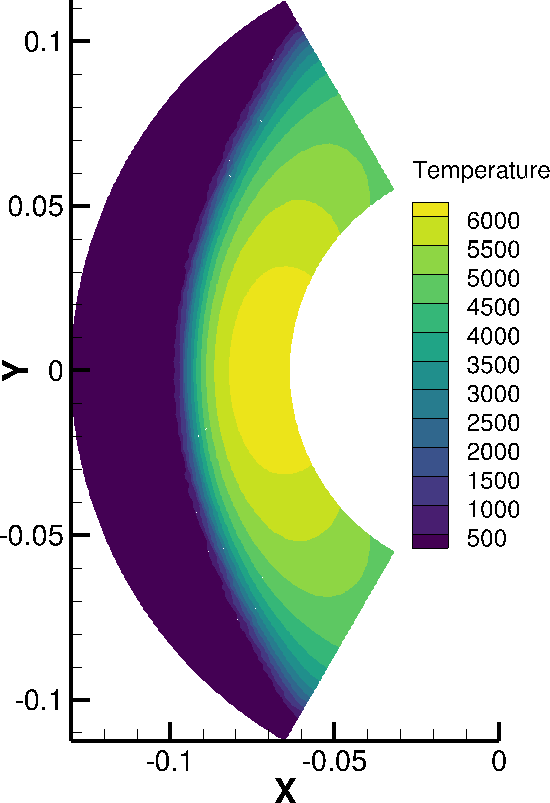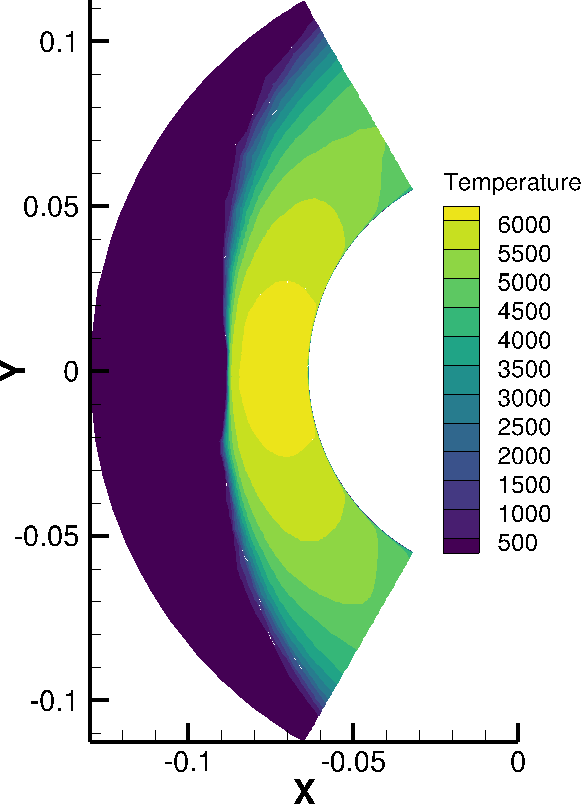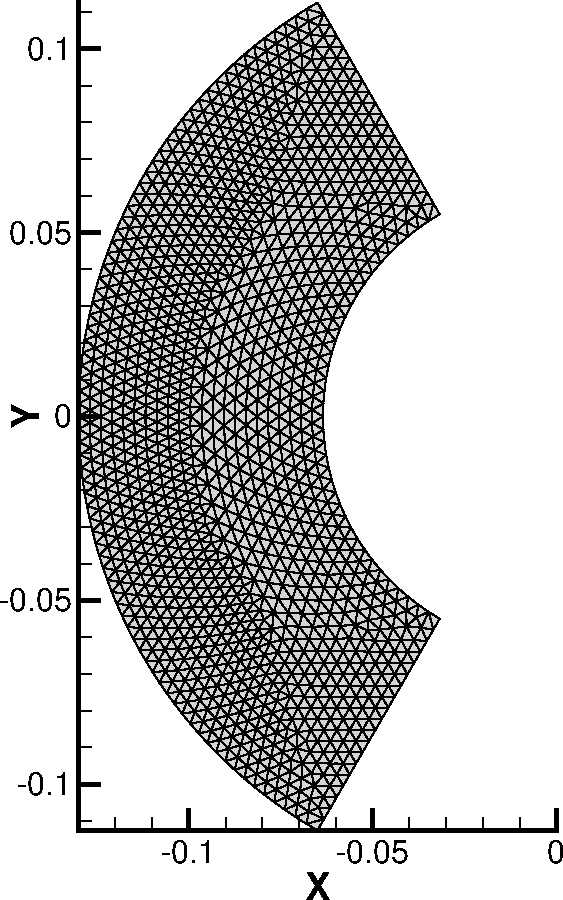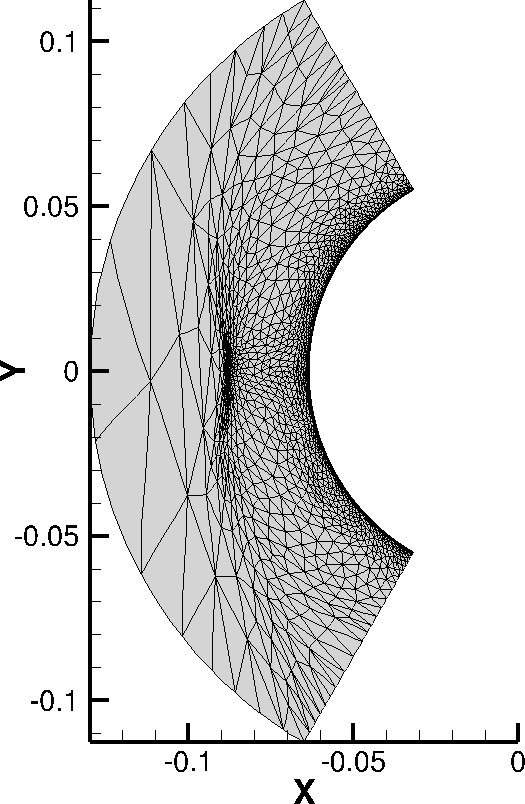Over the next few weeks, Cory Frontin and I are releasing a series of posts giving an overview of the mathematics that underpin output based adaption:

The idea is to present the theory behind how output based adaption works, without going overboard on the mathematics. We’re trying to keep the posts bite-sized, at about 5-10 minute reads each. We'll start with how to mathematically describe the way many physical systems change in time (which includes how fluids move), before moving on to how we can describe the errors from approximately solving this class of problems. Then we’ll finish up with a few posts on a certain class of mesh generation methods, and how our output adaptive algorithm works end-to-end. We’re going to assume a base level of comfort with concepts and notation from integral and differential calculus. We will also be using some notation from more advanced mathematics, but these will be described and motivated as they are introduced.

Before getting into the mathematics, I thought I would share a result from my research as a quick motivation for output adaptive simulation. Let's imagine we have cylinder in a Mach 20 flow. If we were interested in how hot the cylinder would get, then we need to predict the heat flux over the surface of the cylinder. One way to do this is to solve the equations governing fluid dynamics over the cylinder and extract the heat flux from that solution.

Below I have two simulations of part of a 2D slice of the cylinder. In both cases, the surface of the cylinder is the curved boundary on the right hand side, and the Mach 20 air runs left-to-right. The difference in the two simulations is that the result on the left is from the first step of our adaptive algorithm, and the solution on the right is from the last step.Using output based adaption, we can start with the low quality solution on the left, where we're completely missing how the temperature varies near the surface of the cylinder. Then interate (in a completly automated fashion) until we end up with a much higher quality solution like the solution on the right.

The only change that the output adaptive algorithm makes is to the underlying mesh on which we solve the problem. The grid has been made finer, both at the shock centerline (approximately in the middle of the domain) and at the surface of the cylinder. These changes are what result in the higher quality solution.Any questions or comments? Feel free to reach out to me on LinkedIn or Twitter and let me know!